Next: Limb Extraction Up: Mouth Localization Previous: Mouth Localization

## Horizontal Symmetry Projection

Once the symmetry points have been computed for dark symmetry at the appropriate scale, we project the symmetry points along the horizontal. There is no need to consider non-horizontal symmetry points since the mouth is mainly a horizontally oriented limb. The symmetry maps derived by Equationare projected using Equation. Recall that we compute only 8 values of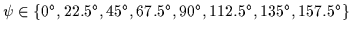(where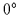is aligned with the horizontal).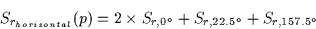(3.2)

Thus, the 4 dimensional symmetry data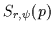is reduced to 3 dimensions in Srhorizontal(p). The projected symmetry (or axial symmetry) maps for r=1 to r=6 are displayed in Figure. These maps are derived from the rotated intensity image in Figure(c).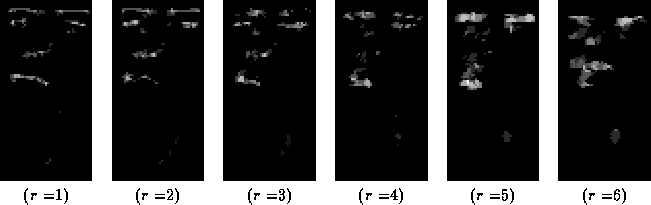The 6 scales (r=1 to r=6) form our axial symmetry scale-space. The scale or r represents the vertical thickness of the horizontal symmetries detected in the image. A thin, closed mouth usually would generate a line of symmetry points at r=1. An open mouth, on the other hand, will generate a cloud of points at a larger r within its center. An open mouth's extremities taper off (since it is closed on both ends) regardless of its size. Thus, the mouth's extremities will appear as clouds at small r.

Note that the symmetry points in Figureare also blurred horizontally with a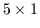Gaussian window to improve connectivity and reduce small gaps. This allows us to use a linking procedure that connects the discrete points in the maps to form connected structures.Next: Limb Extraction Up: Mouth Localization Previous: Mouth Localization
Tony Jebara
2000-06-23